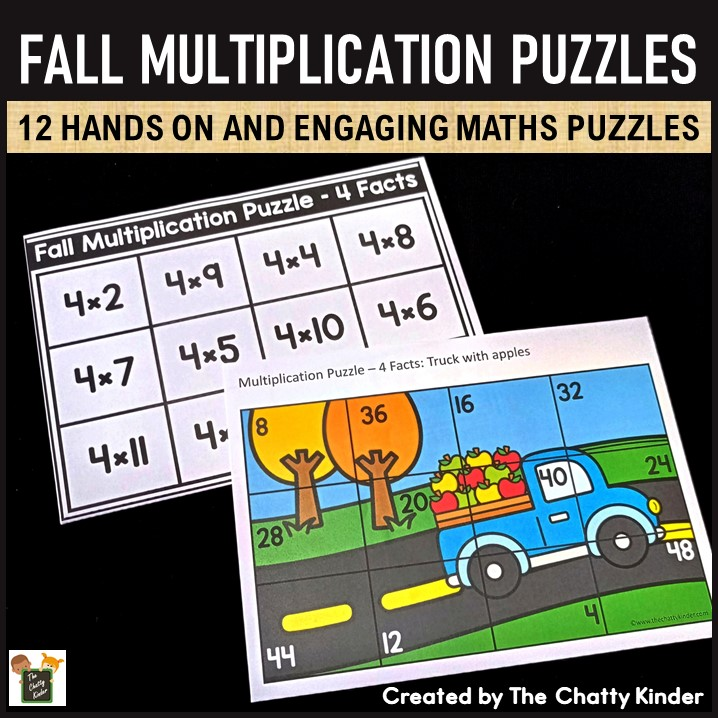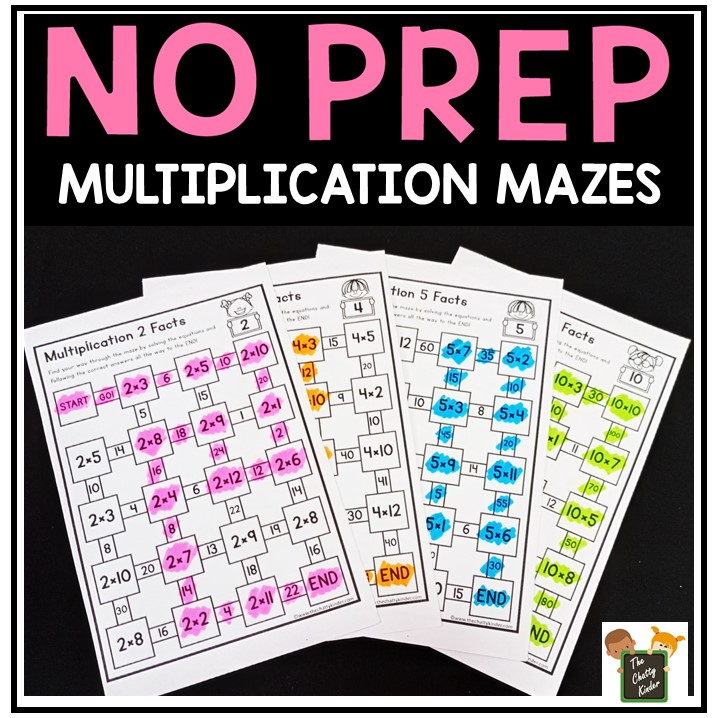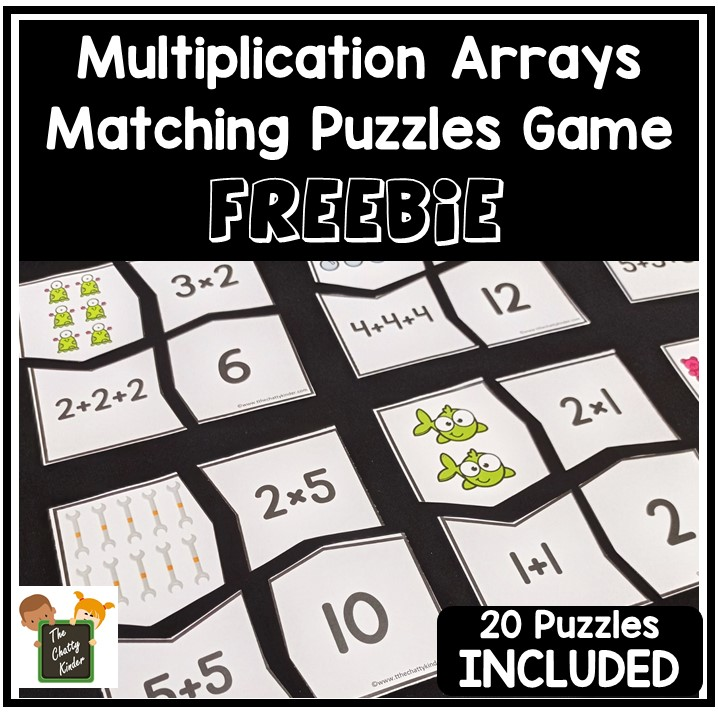# Featured Multiplication and Division## Fall Multiplication Puzzles

Looking for a fun way to practice multiplication this fall? Check out these FREE multiplication puzzles for some fall fun. These FREE Fall fun multiplication puzzles are easy to prep and learners can learn and have fun at the same time. Simple download the fall fun multiplication puzzles printables pdf file and great ready make learning multiplication for 2nd grade, 3rd grade,…## Multiplication Fact Booklets (Repeated Addition of Equal Groups)

Looking for a fun and easy way to introduce multiplication to your learners? Today, I am sharing these low prep multiplication fact booklets. These were extremely useful when I was teaching multiplication. For me as a general rule, maths needs to be understood via concepts and visual aids first. Once the child is familiar with…## No Prep Multiplication Mazes Free Printable

Looking for a fun way to practice those tricky multiplication facts? Grab these No Prep Multiplication Mazes FREE printable to spice things up a little bit! Students will have a blast solving the facts and working their way through the maze. No Prep Multiplication Mazes Free Printable Once students have understood the concept of multiplication,…## Multiplication Arrays: Printable Puzzle Games

What are Math Arrays? Math arrays are an arrangement of objects, pictures, or numbers in rows and columns so that they follow a specific pattern. It is most commonly used as a visual tool for teaching multiplication and division to kids. What are Multiplication Arrays? A multiplication array is simply an arrangement of shapes. objects or pictures in…